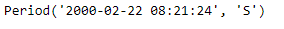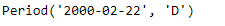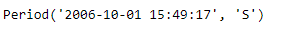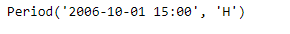# Python | Pandas Period.asfreq

• Last Updated : 06 Jan, 2019

Python is a great language for doing data analysis, primarily because of the fantastic ecosystem of data-centric python packages. Pandas is one of those packages and makes importing and analyzing data much easier.

Pandas` Period.asfreq()` function is used to convert Period to desired frequency, either at the start or end of the interval.

Attention geek! Strengthen your foundations with the Python Programming Foundation Course and learn the basics.

To begin with, your interview preparations Enhance your Data Structures concepts with the Python DS Course. And to begin with your Machine Learning Journey, join the Machine Learning - Basic Level Course

Syntax : Period.asfreq()

Parameters :
freq : string
how : Start or end of the timespan

Return : resampled : Period

Example #1: Use `Period.asfreq()` function to change the frequency of the given period from ‘Seconds’ to ‘Days’

 `# importing pandas as pd``import` `pandas as pd`` ` `# Create the Period object``prd ``=` `pd.Period(freq ``=``'S'``, year ``=` `2000``, month ``=` `2``, day ``=` `22``,``                        ``hour ``=` `8``, minute ``=` `21``, second ``=` `24``)`` ` `# Print the Period object``print``(prd)`

Output :Now we will use the `Period.asfreq()` function to change the frequency of the prd object to ‘Daily frequency’.

 `# change the frequency``prd.asfreq(freq ``=``'D'``)`

Output :As we can see in the output, the `Period.asfreq()` function has successfully changed the frequency of the given object to the desired frequency.

Example #2: Use `Period.asfreq()` function to change the frequency of the given period from ‘Seconds’ to ‘Hours’

 `# importing pandas as pd``import` `pandas as pd`` ` `# Create the Period object``prd ``=` `pd.Period(freq ``=``'S'``, year ``=` `2006``, month ``=` `10``, ``               ``hour ``=` `15``, minute ``=` `49``, second ``=` `17``)`` ` `# Print the object``print``(prd)`

Output :Now we will use the `Period.asfreq()` function to change the frequency of the prd object to ‘hourly frequency’.

 `# change the frequency``prd.asfreq(freq ``=``'H'``)`

Output :As we can see in the output, the `Period.asfreq()` function has successfully changed the frequency of the given object to the desired frequency.

My Personal Notes arrow_drop_up